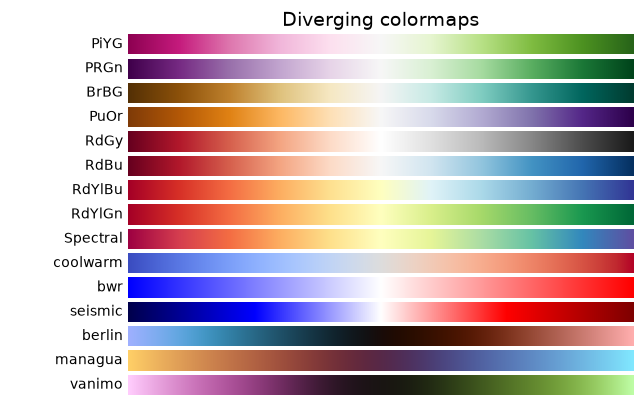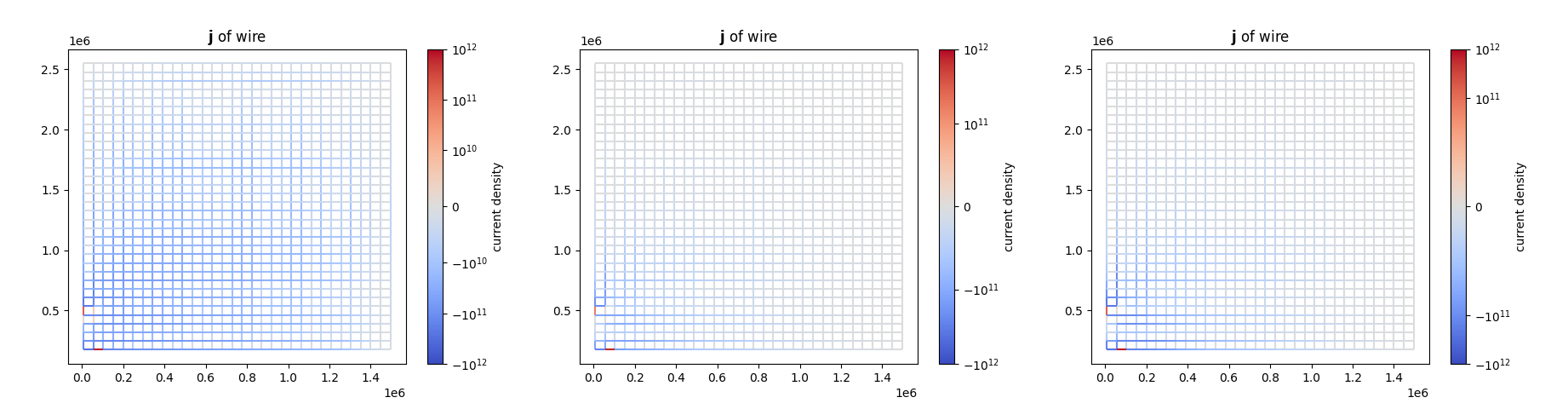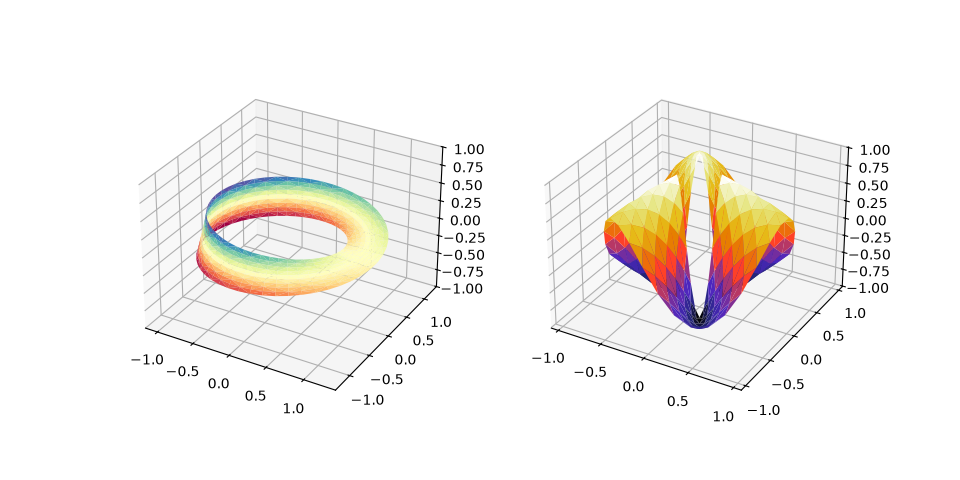# matlbpotlib trick

## 1. Figure

### 1.1. 分辨率

plt.figure(1)
# <Figure size 640x480 with 0 Axes>
plt.figure(1, dpi=300)
# <Figure size 1920x1440 with 0 Axes>
plt.figure(3, dpi=300, figsize=(3,4))
# <Figure size 900x1200 with 0 Axes>


## 2. color

### 2.1. colormap 色表### 2.2. colorbar 色条

fig.colorbar(mappable, ax=ax, location='right', anchor=(0, 0.3), shrink=0.7)


anchor(float, float), optional The anchor point of the colorbar axes. Defaults to (0.0, 0.5) if vertical; (0.5, 1.0) if horizontal.

### 2.3. Colormap Normalization 色表映射正规化

matplotlib真是太神奇辣！这是我觉得最好用的功能，没有之一（想到我之前还手动写 norm = plt.Normalize(norm_lim, -norm_lim) 这种代码就流泪）。

#### 2.3.1. Centered 居中

pc = ax2.pcolormesh(Z, norm=colors.CenteredNorm(), cmap=cmap)


#### 2.3.2. Symmetric logarithmic 居中的对数

pcm = ax.pcolormesh(X, Y, Z,
norm=colors.SymLogNorm(linthresh=0.03, linscale=0.03,
vmin=-1.0, vmax=1.0, base=10),


linscale这个东西就比较神秘：

The size of this range in the colormap is set by linscale. When linscale == 1.0 (the default), the space used for the positive and negative halves of the linear range will be equal to one decade in the logarithmic range.

# 从左到右对应下面的三个正规化bar
norm1 = colors.SymLogNorm(
linthresh=10**10, base=10, vmin=-10**12, vmax=10**12, linscale=1
)
norm2 = colors.SymLogNorm(
linthresh=10**11, base=10, vmin=-10**12, vmax=10**12, linscale=1
)
norm3 = colors.SymLogNorm(
linthresh=10**11, base=10, vmin=-10**12, vmax=10**12, linscale=2
)norm1和norm2有参数linscale=1，可以看到刻度都是均匀的；而norm3有参数linscale=2，0到$\plusmn 10^{11}$的间距就是对数轴那边的两倍。

## 3. 2D

### 3.1. 如何在 matplotlib 中绘制二维结构化网格

How to plot a 2d structured mesh in matplotlib

import numpy as np
import matplotlib.pyplot as plt
from matplotlib.collections import LineCollection

x, y = np.meshgrid(np.linspace(0,1, 11), np.linspace(0, 0.6, 7))

segs1 = np.stack((x[:,[0,-1]],y[:,[0,-1]]), axis=2)
segs2 = np.stack((x[[0,-1],:].T,y[[0,-1],:].T), axis=2)

plt.autoscale()
plt.show()


### 3.2. 给网格（线段）按值上色

Multicolored lines

lc = LineCollection(segments, cmap='viridis', norm=norm)
lc.set_array(dydx)


### 3.4. 画圆

circle = plt.Circle((1, 1), 0.2, color='g', clip_on=False)


### 3.5. 确定图层

circle = Circle(
(info["xpos"], info["ypos"]),
color=color,
fill=False,
zorder=10000,
)


circ.append(Circle ((1,1), 0.2))
coll=PatchCollection(circ, zorder=10)


## 4. 3D

### 4.1. 使用XYZ一维数组/散点绘制三维平面

surface plots in matplotlib